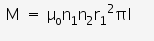# Derive the expression for the mutual inductance of two long coaxial

Derive the expression for the mutual inductance of two long coaxial solenoids of same length l having radii r1 and r2 (r2>r1 and l>>r2). is it the derivation for mutual inductance due to two solenoids?

Suppose a current i is passed through the inner solenoid
S1. A magnetic fieldis produce inside S1
whereas field outside it is zero.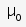is permeability of vaccum.
Let n1 and n2 be the no of turns it each solenoid of equal length l.

Therefore, flux through each turn of solenoid S2 is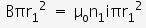The total flux through all the turns in a length l of S2 is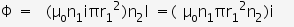And we know that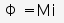where M is the mutual inductance.
Therefore mutual inductance of two long coaxial solenoids of same lenth is